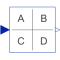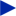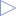# StateSpace

Linear state space system# Information

This information is part of the Modelica Standard Library maintained by the Modelica Association.

The State Space block defines the relation between the input u and the output y in state space form:

```
der(x) = A * x + B * u
y  = C * x + D * u
```

The input is a vector of length nu, the output is a vector of length ny and nx is the number of states. Accordingly

```        A has the dimension: A(nx,nx),
B has the dimension: B(nx,nu),
C has the dimension: C(ny,nx),
D has the dimension: D(ny,nu)
```

Example:

```     parameter: A = [0.12, 2;3, 1.5]
parameter: B = [2, 7;3, 1]
parameter: C = [0.1, 2]
parameter: D = zeros(ny,nu)
results in the following equations:
[der(x)]   [0.12  2.00] [x]   [2.0  7.0] [u]
[         ] = [          ]*[    ] + [        ]*[    ]
[der(x)]   [3.00  1.50] [x]   [0.1  2.0] [u]
[x]            [u]
y   = [0.1  2.0] * [    ] + [0  0] * [    ]
[x]            [u]
```

# Parameters (9)

nin Value: size(B, 2) Type: Integer Description: Number of inputs Value: size(C, 1) Type: Integer Description: Number of outputs Value: [1, 0; 0, 1] Type: Real[:,size(A, 1)] Description: Matrix A of state space model (e.g., A=[1, 0; 0, 1]) Value: [1; 1] Type: Real[size(A, 1),:] Description: Matrix B of state space model (e.g., B=[1; 1]) Value: [1, 1] Type: Real[:,size(A, 1)] Description: Matrix C of state space model (e.g., C=[1, 1]) Value: zeros(size(C, 1), size(B, 2)) Type: Real[size(C, 1),size(B, 2)] Description: Matrix D of state space model Value: Modelica.Blocks.Types.Init.NoInit Type: Init Description: Type of initialization (1: no init, 2: steady state, 3: initial state, 4: initial output) Value: zeros(nx) Type: Real[nx] Description: Initial or guess values of states Value: zeros(ny) Type: Real[ny] Description: Initial values of outputs (remaining states are in steady state if possible)

# Outputs (1)

x Type: Real[size(A, 1)] Description: State vector

# Connectors (2)

u yType: RealInput[nin] Description: Connector of Real input signalsType: RealOutput[nout] Description: Connector of Real output signals